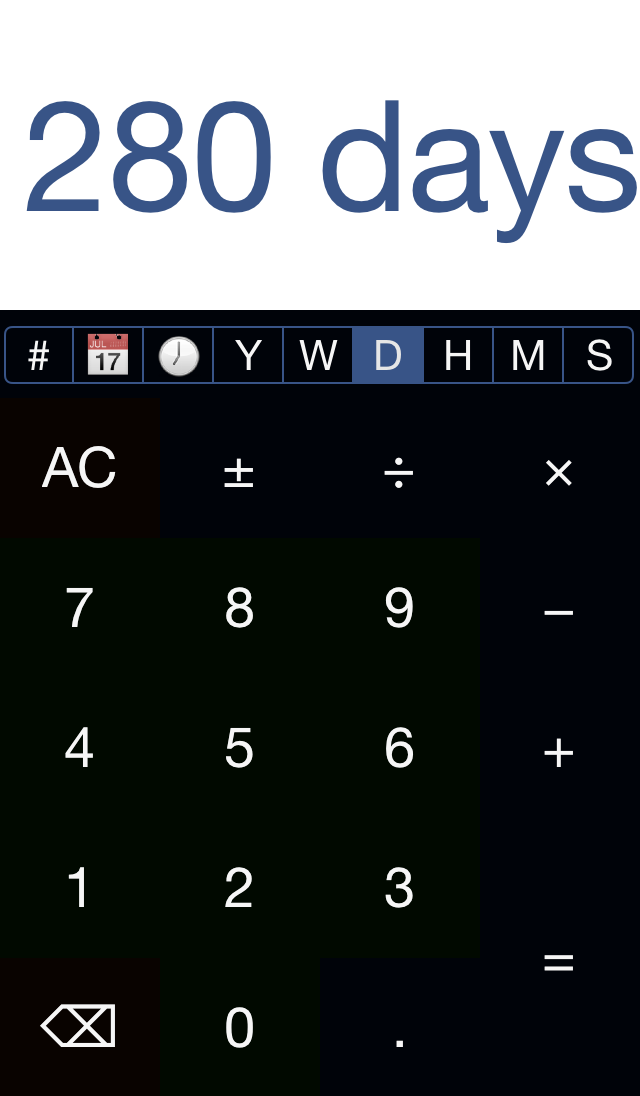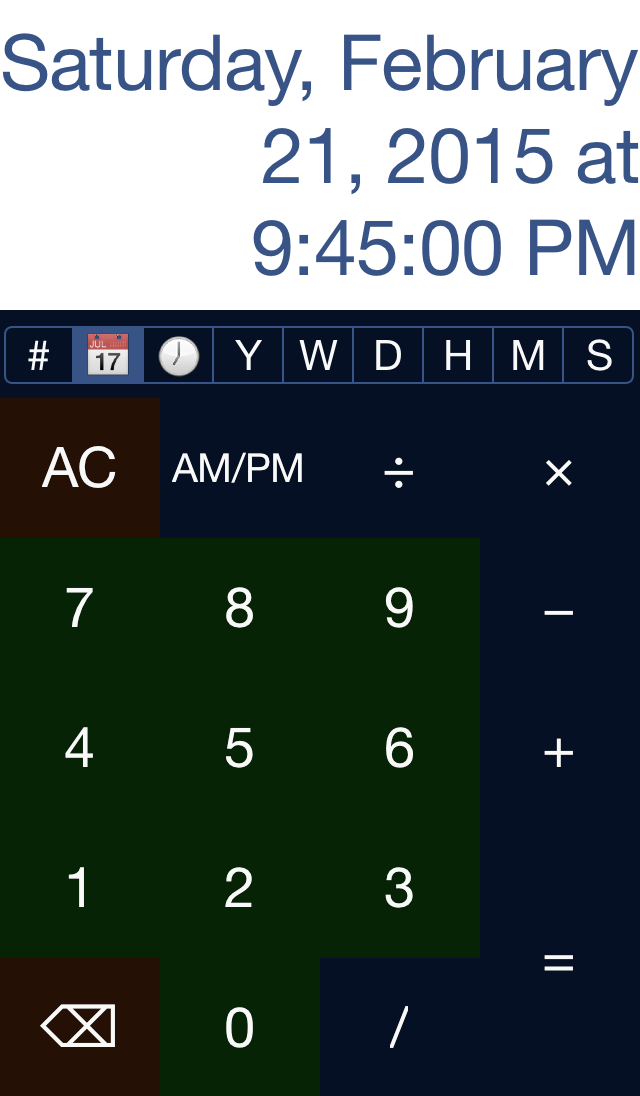Crunch Time: Power
Crunch time.
A Time & Date Calculator + Unit Converter× Calculate dates, times, years, days, hours, minutes, & seconds.

− Simple design, powerful functionality

÷ Includes addition, subtraction, multiplication, division, and more

+ Works just like you would expect a calculator to work

• Easily and rapidly input dates, times, or both
• Perform calculations in the units of your choice, then convert the answer to any other unit
• Choose how precise your answers are
• Choose how detailed your dates are
• Track how long until an event or how long since one occurred
• If I ran a 5k in 20:09, then what was my pace?: 20:09 ÷ 3.1 = 6:30.
• What if I took about 7 seconds off my pace, then how long would it take to complete my workout?: 6:30 - 7 = 6:23 × 3.1 = 19:48.
• If I do something for 35 minutes 12 times, how much total time did it take?: 35:00 × 12 = 7h 00m 00s.
• How many minutes is 8 hours?
• How many seconds?
• How long has it been since we first met?
• When is 6 weeks from today?
• How many seconds in 30 years?
• How many days is 1,000,000 seconds?
• What about hours?
• You, too can answer these and other time-related questions with CrunchTime

Crunch time with CrunchTime.